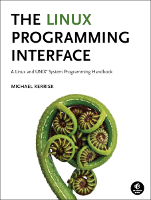This is threads/thread_lock_speed.c, an example to accompany the book, The Linux Programming Interface.

This file is not printed in the book; it is a supplementary file for Chapter 33.

In the listing below, the names of Linux system calls and C library functions are hyperlinked to manual pages from the Linux man-pages project, and the names of functions implemented in the book are hyperlinked to the implementations of those functions.• Distribution version

## Function list (Bold in this list means a function is not static)

```/* thread_lock_speed.c

This program employs POSIX threads that increment the same global
variable, synchronizing their access using either a mutex or a spinlock.
Command-line arguments allow the user to specify:

* The number of threads that will increment the global variable.
* The number of "outer loops" that each thread will execute using
the lock/unlock APIs (either mutexes or spin locks).
* The number "inner loops" executed for each "outer loop" step.
Each inner loop iteration increments the global variable by 1.

the global variable. Specifying the "-s" option causes spin locks

The idea is to vary the number of threads and number of inner loops
while using time(1) to measure the real and CPU time consumed by the
program. In some scenarios (e.g., many threads, large "inner loop"
values), mutexes will perform better, while in others (few threads,
small "inner loop" value), spin locks are likely to be better.
*/
#include <stdbool.h>
#include "tlpi_hdr.h"

static volatile int glob = 0;
static bool useMutex;
static int numOuterLoops;
static int numInnerLoops;
```
```static void *
{
int s;

for (int j = 0; j < numOuterLoops; j++) {
if (useMutex) {
if (s != 0)
} else {
if (s != 0)
}

for (int k = 0; k < numInnerLoops; k++)
glob++;

if (useMutex) {
if (s != 0)
} else {
if (s != 0)
}
}

return NULL;
}
```
```static void
usageError(char *pname)
{
fprintf(stderr,
"[num-inner-loops [num-outer-loops]]\n", pname);
fprintf(stderr,
"    -q   Don't print verbose messages\n");
fprintf(stderr,
"    -s   Use spin locks (instead of the default mutexes)\n");
exit(EXIT_FAILURE);
}
```
```int
main(int argc, char *argv[])
{
/* Prevent runaway/forgotten process from burning up CPU time forever */

alarm(120);         /* Unhandled SIGALRM will kill process */

useMutex = true;
bool verbose = true;
int opt;
while ((opt = getopt(argc, argv, "qs")) != -1) {
switch (opt) {
case 'q':
verbose = false;
break;
case 's':
useMutex = false;
break;
default:
usageError(argv);
}
}

if (optind >= argc)
usageError(argv);

numInnerLoops = (optind + 1 < argc) ? atoi(argv[optind + 1]) : 1;
numOuterLoops = (optind + 2 < argc) ? atoi(argv[optind + 2]) : 10000000;

if (verbose) {
printf("Using %s\n", useMutex ? "mutexes" : "spin locks");
printf("\tthreads: %d; outer loops: %d; inner loops: %d\n",
}

errExit("calloc");

int s;

if (useMutex) {
if (s != 0)
} else {
if (s != 0)
}

for (int j = 0; j < numThreads; j++) {
if (s != 0)
}

for (int j = 0; j < numThreads; j++) {